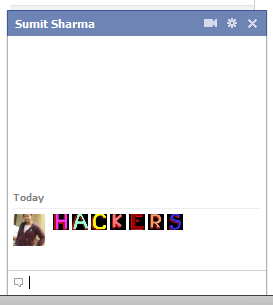## Saturday, 7 April 2012

### Colorful Alphabets For Facebook ChatHello to Everyone, Today I have a post for Facebook fans. We normally chat with simple alphabets but now you can use colorful alphabets to make chat so interesting.

Now as you can see how beautiful it looks when you will this kind of message to your friends. So now if you like this thing and you wanna start a colorful alphabets emoticon chatting than you wanna use below code for different alphabets mentioned. SO whenever you paste code for any alphabet and you hit enter you will get colorful alphabets like above. So copy codes from below and start chatting.

Below is the list:

[] = A
[] = B
[] = C
[] = D
[] = E
[] = F
[] = G
[] = H
[] = I
[] = J
[] = K
[] = L
[] = M
[] = N
[] = O
[] = P
[] = Q
[] = R
[] = S
[] = T
[] = U
[] = V
[] = W
[] = X
[] = Y
[]  = Z

So Now try it out...........